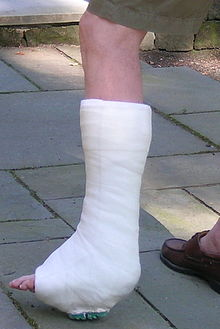# 5.11: Formula Mass: The Mass of a Molecule or Formula Unit

Skills to Develop

• To determine the formula mass of an ionic compound.

One skill needed in future chapters is the ability to determine the mass of the formula of an ionic compound. This quantity is called the formula mass. The formula mass is obtained by adding the masses of each individual atom in the formula of the compound. Because a proper formula is electrically neutral (with no net electrons gained or lost), the ions can be considered atoms for the purpose of calculating the formula mass.

Let us start by calculating the formula mass of sodium chloride (NaCl). This formula mass is the sum of the atomic masses of one sodium atom and one chlorine atom, which we find from the periodic table; here, we use the masses to two decimal places:

Na:    22.99 amu

Cl:   +35.34 amu

Total: 58.44 amu

To two decimal places, the formula mass of NaCl is 58.44 amu.

When an ionic compound has more than one anion or cation, you must remember to use the proper multiple of the atomic mass for the element in question. For the formula mass of calcium fluoride (CaF2), we must multiply the mass of the fluorine atom by 2 to account for the two fluorine atoms in the chemical formula:

Ca: 1 x 40.08 =   40.08 amu

F:   2 x 19.00 = +38.00 amu

Total =   78.08 amu

The formula mass of CaF2 is 78.08 amu.

For ionic compounds with polyatomic ions, the sum must include the number and mass of each atom in the formula for the polyatomic ion. For example, potassium nitrate (KNO3) has one potassium atom, one nitrogen atom, and three oxygen atoms:

K: 1 x 39.10 =   39.10 amu

N: 1 x 14.00 = +14.00 amu

O: 3 x 16.00 = +48.00 amu

Total = 101.10 amu

The formula mass of KNO3 is 101.10 amu.

Potassium nitrate is a key ingredient in gunpowder and has been used clinically as a diuretic.

When a formula contains more than one polyatomic unit in the chemical formula, as in Ca(NO3)2, do not forget to multiply the atomic mass of every atom inside the parentheses by the subscript outside the parentheses. This is necessary because the subscript refers to the entire polyatomic ion. Thus, for Ca(NO3)2, the subscript 2 implies two complete nitrate ions, so we must sum the masses of two (1 × 2) nitrogen atoms and six (3 × 2) oxygen atoms, along with the mass of a single calcium atom:

Ca:  1 x 40.08 =   40.08 amu

N:   2 x 14.00 = +28.00 amu

O:   6 x 16.00 = +96.00 amu

Total = 164.08 amu

The key to calculating the formula mass of an ionic compound is to correctly count each atom in the formula and multiply the atomic masses of its atoms accordingly.

Example $$\PageIndex{1}$$

Use the atomic masses (rounded to two decimal places) from the inside cover of this book to determine the formula mass for each ionic compound.

1. FeCl3
2. (NH4)3PO4

SOLUTION

a.

Fe: 1 x 55.85 =    55.85 amu

Cl: 1 x 35.45 = +106.35 amu

________________________

Total = 162.20 amu

The formula mass of FeCl3 is 162.2 amu.

b. When we distribute the subscript 3 through the parentheses containing the formula for the ammonium ion, we see that we have 3 nitrogen atoms and 12 hydrogen atoms. Thus, we set up the sum as follows:

N: 3 x 14.00 =  42.00 amu

H: 12 x 1.00 = +12.00 amu

P: 1 x 30.97 = +30.97 amu

O: 4 x 16.00 = +64.00 amu

Total = 148.97 amu

The formula mass for (NH4)3PO4 is 149.0 amu.

Exercise $$\PageIndex{1}$$

Use the atomic masses (rounded to two decimal places) from the inside cover of this book to determine the formula mass for each ionic compound.

1. TiO2
2. AgBr
3. Au(NO3)3
4. Fe3(PO4)2

a.  79.87 amu

b.  187.77 amu

c.  383.0 amu

To Your Health: Hydrates

Some ionic compounds have water (H2O) incorporated within their formula unit. These compounds, called hydrates, have a characteristic number of water units associated with each formula unit of the compound. Hydrates are solids, not liquids or solutions, despite the water they contain.

To write the chemical formula of a hydrate, write the number of water units per formula unit of compound after its chemical formula. The two chemical formulas are separated by a vertically centered dot. The hydrate of copper(II) sulfate has five water units associated with each formula unit, so it is written as CuSO4•5H2O. The name of this compound is copper(II) sulfate pentahydrate, with the penta- prefix indicating the presence of five water units per formula unit of copper(II) sulfate.Calcium sulfate hemihydrate (CaSO4•½H2O), or plaster of Paris, is used to make casts to immobilize broken bones until they heal. Figure used with permisison from Wikipedia

Hydrates have various uses in the health industry. Calcium sulfate hemihydrate (CaSO4•½H2O), known as plaster of Paris, is used to make casts for broken bones. Epsom salt (MgSO4•7H2O) is used as a bathing salt and a laxative. Aluminum chloride hexahydrate is an active ingredient in antiperspirants. The accompanying table lists some useful hydrates.

Table $$\PageIndex{1}$$: Names and Formulas of Some Widely Used Hydrates
Formula Name Uses
AlCl3•6H2O aluminum chloride hexahydrate antiperspirant
CaSO4•½H2O calcium sulfate hemihydrate (plaster of Paris) casts (for broken bones and castings)
CaSO4•2H2O calcium sulfate dihydrate (gypsum) drywall component
CoCl2•6H2O cobalt(II) chloride hexahydrate drying agent, humidity indicator
CuSO4•5H2O copper(II) sulfate pentahydrate fungicide, algicide, herbicide
MgSO4•7H2O magnesium sulfate heptahydrate (Epsom salts) laxative, bathing salt
Na2CO3•10H2O sodium carbonate decahydrate (washing soda) laundry additive/cleane

### KEY TAKEAWAY

• Formula masses of ionic compounds can be determined from the masses of the atoms in their formulas.

### Contributors

• Anonymous

• Henry Agnew (UC Davis)